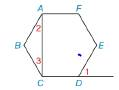Chapter 2.5, Problem 28E### Elementary Geometry for College St...

6th Edition
Daniel C. Alexander + 1 other
ISBN: 9781285195698

#### Solutions

Chapter
Section### Elementary Geometry for College St...

6th Edition
Daniel C. Alexander + 1 other
ISBN: 9781285195698
Textbook Problem
3 views

# Given: Regular hexagon ABCDEF with diagonal A ¯ C ¯ and exterior ∠ 1 . Prove: m ∠ 2+m ∠ 3 = m ∠ 1To determine

To prove:

m2+m3=m1

Explanation

Given:

The following figure shows the given diagram.

Figure (1)

EDC is supplementary to 1 as shown in given figure.

Calculation:

The completed proof is shown in the following table.

 Proof Statements Reasons 1. The ∠EDC is supplementary to ∠1 1. Given 2.m∠1+m∠EDC=180° 2. The sum of measures of two supplementary angles is 180° 3.m∠B+m∠2+m∠3=180° 3. The sum of measures of four angles in a quadrilateral is 180°. 4. Regular hexagon ABCDEF 4. Given 5.m∠B=m∠EDC 5. The measures of interior angles in a regular hexagon will be same. 6.m∠2+m∠3=m∠1 6. Solve (2), (3), and (5)

Conclusion:

Thus, the completed proof is shown in the following table

### Still sussing out bartleby?

Check out a sample textbook solution.

See a sample solution

#### The Solution to Your Study Problems

Bartleby provides explanations to thousands of textbook problems written by our experts, many with advanced degrees!

Get Started

#### Find more solutions based on key concepts